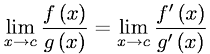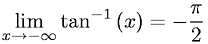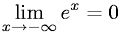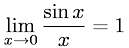Calculus > LimitsShowing results 1 to 7 of 7, on page 1 of 1
 DESCRIPTION EQUATION L'Hopital's RuleLimit of Arctangent X as X Approaches InfinityLimit of Arctangent X as X Approaches Negative InfinityLimit of e to the X power as X Approaches Negative InfinityLimit of One over X to the nth PowerLimit of Sine X over X as X Approaches ZeroLimit of Tangent X over X as X Approaches Zero